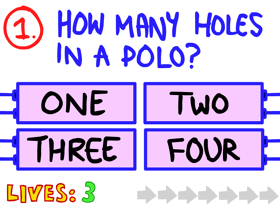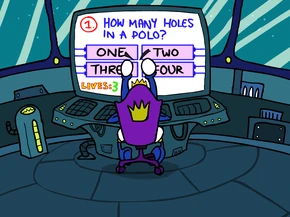## FANDOM

478 Pages

 The Impossible Quiz << Final Beta Question Question 1 Next Question >>
Question 1Difficulty

Easy

Reference(s)

Question 1 (TIQD)

Question 1 of the original Impossible Quiz is the very first question in the series that was taken from The Impossible Quiz Demo (where it was also the first question). It reads "How many holes in a polo?", with the possible answers being all numbers from one to four, written in letters.The cameo of this question in the intro of TIQB, Chapter 2.

The answer to the question is "Four", since it's talking about the holes in the letters that form the words "a polo". There is one hole each in the letters A, P, and the two O's.

## Trivia

• This is also the first question in a series of recurring questions in across the Impossible Quiz series that ask you "How many holes in..." a seemingly random item. These questions include number 100 in this Quiz, number 30 in The Impossible Quiz 2 and lastly number 105 in The Impossible Quiz Book (Chapter 3).
• During the introductory cutscene of The Impossible Quiz Book, Chapter 2, the Phlovomite King can be seen in his spaceship playing the first Quiz. He seems to be struggling with this particular question, which is displayed on his screen right before he gets a Game Over, much to his annoyance.
• One of the tips of the day in The Impossible Quiz 2 reference this question.